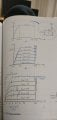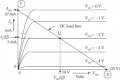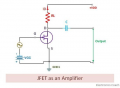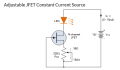# Linear FET amplification?

Status
Not open for further replies.

#### PatrickMalarkey

Joined Oct 2, 2021
111
I've been reading a textbook section about JFETs and their amplification, which leaves me with the rather confounded question: Is FET amplification output, linear to it's gate input (voltage), especially throughout it's "constant-current" regions? If not, what is the reason for graphing that region beyond pinch off, captioned "constant-current region"? Please find a photo from my book.#### MrChips

Joined Oct 2, 2009
25,948
It is useful to draw a load line on the I-V transistor characteristic curves.The load line represents the load resistor RL from the VDD supply line to the drain of the transistor.

Point-X on the graph indicates the point where the transistor is fully turned off. IDS is 0 and the VDS is VDD (the supply voltage, 20V in this example).

Point-Y on the graph indicates the point where the transistor is fully turned on. VDS is 0 and VR (voltage across RL) is VDD. By applying Ohm's Law, IDS = VR/ RL

In other words, the slope of the load line = IDS / VR = 1 / RL

Working backwards = RL = VDD / Imax = 20V / 10mA = 2kΩ

The important point to note is that the operating point of the transistor must lie on the load line at all times.
Knowing this, we can select a suitable Q-point (quiescent point or DC bias point). We can also determine the voltage gain of this amplifier stage.

For example, the graph shows a suitable Q-point where the VGS bias voltage is -2V.
Now if we apply a signal of 1V amplitude to the gate, i.e. a signal swinging from -3V to -1V, we can follow VDS voltage on the x-axis.
Let us estimate that at VGS = -1V, VDS = 3V
and at VGS = -3V, VDS = 15V

The voltage gain is (15 - 3) / (-3 +1) = 12/(-2) = -6

The linearity or non-linearity of this amplifier stage depends on how equally spaced are the VGS lines.
If the -3V to -2V lines are spaced the same as the -2V and -1V lines, then we can expect this amplifier stage to be linear.

For the example analysis given above, we can conclude that this stage is non-linear when the input signal spans such a wide range from -3V to -1V.

A Q-point of VGS = -2.5V and a lower amplitude input signal might produce an output that is closer to being linear.

•PeteHL

#### PatrickMalarkey

Joined Oct 2, 2021
111
It is useful to draw a load line on the I-V transistor characteristic curves.

View attachment 255258

View attachment 255259

The load line represents the load resistor RL from the VDD supply line to the drain of the transistor.

Point-X on the graph indicates the point where the transistor is fully turned off. IDS is 0 and the VDS is VDD (the supply voltage, 20V in this example).

Point-Y on the graph indicates the point where the transistor is fully turned on. VDS is 0 and VR (voltage across RL) is VDD. By applying Ohm's Law, IDS = VR/ RL

In other words, the slope of the load line = IDS / VR = 1 / RL

Working backwards = RL = VDD / Imax = 20V / 10mA = 2kΩ

The important point to note is that the operating point of the transistor must lie on the load line at all times.
Knowing this, we can select a suitable Q-point (quiescent point or DC bias point). We can also determine the voltage gain of this amplifier stage.

For example, the graph shows a suitable Q-point where the VGS bias voltage is -2V.
Now if we apply a signal of 1V amplitude to the gate, i.e. a signal swinging from -3V to -1V, we can follow VDS voltage on the x-axis.
Let us estimate that at VGS = -1V, VDS = 3V
and at VGS = -3V, VDS = 15V

The voltage gain is (15 - 3) / (-3 +1) = 12/(-2) = -6

The linearity or non-linearity of this amplifier stage depends on how equally spaced are the VGS lines.
If the -3V to -2V lines are spaced the same as the -2V and -1V lines, then we can expect this amplifier stage to be linear.

For the example analysis given above, we can conclude that this stage is non-linear when the input signal spans such a wide range from -3V to -1V.

A Q-point of VGS = -2.5V and a lower amplitude input signal might produce an output that is closer to being linear.
I followed that example to the extent "and at Vgs=-3V, Vds=15V." Prior to those values Vgs=-1V with a Vds=3V, presumably with a gate biasing voltage of -2 V. Voltage between the source and drain, in my understanding, is the fixed supply voltage, not 3V and 15V, just one or the other even if input voltages we're additive with the supply voltage. The discussion of using a load line in determining linearity is note worthy, but my thought has been that if the Q point and signal swing is somewhere along the "constant current region" amplification is linear, or nearly so to the input signal???

#### Ian0

Joined Aug 7, 2020
4,899
Is FET amplification output, linear to it's gate input (voltage), especially throughout it's "constant-current" regions?
No - it's not linear. You textbook shows an "idealised" FET. In reality, those lines get closer together towards the bottom of the graph, because the Vgs/Id relationship is square-law, and I think this was mentioned a couple of weeks ago in a different post.
See page 2 of this datasheet for a real FET, and it is the same example that I quoted last time you asked. When you draw your load-line, you will find that the relationship between Vgs and Id is no longer a straight line like it was in your text book.

#### PatrickMalarkey

Joined Oct 2, 2021
111
No - it's not linear. You textbook shows an "idealised" FET. In reality, those lines get closer together towards the bottom of the graph, because the Vgs/Id relationship is square-law, and I think this was mentioned a couple of weeks ago in a different post.
See page 2 of this datasheet for a real FET, and it is the same example that I quoted last time you asked. When you draw your load-line, you will find that the relationship between Vgs and Id is no longer a straight line like it was in your text book.
That certainly explains things, I suppose my book ficticiously provided a simpler graph for ease in understanding. But if graph and numbers aren"t correct then the book is misleading just about as bad as the devil wife.

#### Ian0

Joined Aug 7, 2020
4,899
That certainly explains things, I suppose my book ficticiously provided a simpler graph for ease in understanding. But if graph and numbers aren"t correct then the book is misleading just about as bad as the devil wife.
I think the author is trying to explain Gfs, and that is a lot easier if we assume that Gfs is a constant.
(unfortunately, it isn’t)

#### Audioguru again

Joined Oct 21, 2019
4,539
A Fet's high input impedance is needed at the input of the preamp for an electric guitar. The users and listeners "like" the non-linear distortion.

#### Ian0

Joined Aug 7, 2020
4,899
A Fet's high input impedance is needed at the input of the preamp for an electric guitar. The users and listeners "like" the non-linear distortion.
Not just the guitarists! it’s the same Vgs/Id non-linearity that the golden-ears brigade pay £10000 a go for a 300B single-ended triode amplifier (with transformers wound with silver wire).

•Delta Prime

#### Audioguru again

Joined Oct 21, 2019
4,539
Extremely expensive antique-looking amplifiers are sold today with a transistors or IC circuit and a pair of vacuum tubes glowing on top that are not in the amplifier circuit.

•SamR

#### PatrickMalarkey

Joined Oct 2, 2021
111
I think the author is trying to explain Gfs, and that is a lot easier if we assume that Gfs is a constant.
(unfortunately, it isn’t)
I'm trying to find a way to correct for the non-linearity of JFET amplification. Because of my hopes of using JFETs rather than bipolar transistors. And that due to less attenuation of musical instrument's distinguishing harmonics, by JFETs. I am assuming that JFETs pass those harmonics and bipolar transistors don't. That attenuation might be caused by waveform alteration by the bypass and coupling capacitors used in biasing bipolar amps. Direct coupling of bipolar amp stages might be another way to avoid the alteration, and it's been tried, and I haven't heard anything other than noise troubles arose.

#### Ian0

Joined Aug 7, 2020
4,899
I am assuming that JFETs pass those harmonics and bipolar transistors don't.
I can get lower distortion with bipolar transistors, but that is probably because they have higher Gfs and I use a lot of feedback.
For a single transistor circuit, a JFET design would probably give lower distortion than a bipolar, but it would take a really bad design to attenuate the audible harmonics of musical instruments.
If you are thinking of guitar pickup amplification, rather than microphone amplification, the higher input impedance of the JFET does give it an advantage.
To take an example, the famous Fuzzface circuit is bipolar, it has a very low input impedance, and it removes most of the harmonics (then it adds its own, but that's its purpose)

#### Audioguru again

Joined Oct 21, 2019
4,539
If your speakers are hifi and high quality then they can pass frequencies as high as we can hear which is up to 20kHz. We cannot hear higher harmonics.
We do not understand why you wrongly believe that bipolar transistors attenuate harmonics produced by musical instruments because a bipolar amplifier easily produces frequencies as high as 100kHz or more.
A bipolar amplifier uses a bypass capacitor in its power supply circuit, not in the audio path.
A coupling capacitor blocks DC and very low frequencies, not high frequency harmonics.

#### Papabravo

Joined Feb 24, 2006
18,152
If your speakers are hifi and high quality then they can pass frequencies as high as we can hear which is up to 20kHz. We cannot hear higher harmonics.
We do not understand why you wrongly believe that bipolar transistors attenuate harmonics produced by musical instruments because a bipolar amplifier easily produces frequencies as high as 100kHz or more.
A bipolar amplifier uses a bypass capacitor in its power supply circuit, not in the audio path.
A coupling capacitor blocks DC and very low frequencies, not high frequency harmonics.
It seems almost cruel to destroy a fantasy with such basic facts. Maybe a spoonful of sugar would help the medicine go down.

#### PatrickMalarkey

Joined Oct 2, 2021
111
I can get lower distortion with bipolar transistors, but that is probably because they have higher Gfs and I use a lot of feedback.
For a single transistor circuit, a JFET design would probably give lower distortion than a bipolar, but it would take a really bad design to attenuate the audible harmonics of musical instruments.
If you are thinking of guitar pickup amplification, rather than microphone amplification, the higher input impedance of the JFET does give it an advantage.
To take an example, the famous Fuzzface circuit is bipolar, it has a very low input impedance, and it removes most of the harmonics (then it adds its own, but that's its purpose)
[/

#### sparky 1

Joined Nov 3, 2018
706
In the circle is a symbol DC it is abbreviated and it is assumed that you will know about non conventional symbols for power source.
https://components101.com/articles/electronics-components-and-their-symbols
I will post a non abbreviated symbol (a schematic) of what an adjustable current source might look like below.
There are much better current sources and voltage sources, some very elaborate the schematic would be extensive. maybe confusing.

Linear and non linear review when the book is edited properly for corrections it would be clear.
Capacitors and resistors are linear however transistors and diodes are non-linear on an I/V curve.
A change in base current of a transistor results in new IV curve whereas a diode has only two terminals.
As the base current increases the saturation region decreases slightly.

Discontinuity in the book's logic the label CC region probably needs correction, a brilliant question might be:
Since adjusting current is important what might a jfet CC circuit look like ? and would a 1k pot get the current down enough? (100 uA)
Why not include CC circuit in a corresponding lab so a student could actually build and understand IV curve tracer. (not enough time)
In the assignment draw an IV curve and label it. Looking at the saturation region could some jfets in a constant current circuit maintain
almost constant over a specified voltage range. So then are some jfets almost linear?Last edited:

#### PatrickMalarkey

Joined Oct 2, 2021
111
The acoustic instrument's waveform is a deteriorating waveform in both it's frequency and intensity. And in modern linear amplification the use of passive devices, in shunt with or series with linear biasing resistors, alters that waveform towards a more regular (or basic sinusoidal) waveform. The use of non-linear amplification, without biasing capacitors, or tone control inductors, might offer a solution to that alteration. However non-linear devices such as the FET and MOSFET have intrinsic, semiconductor- capacitances in their P-N junctions that impart an alteration of their own that mimic the true acoustic instrument's sound wave. What is ideal amplification? Maybe a compensated FET whose P-N junction capacitance alters the acoustic qualities of it's amplified signal to match that of an instrument.

#### Ya’akov

Joined Jan 27, 2019
5,559
Amplifiers use negative feedback to eliminate artifacts. I am not at all clear what sort of distortion you are concerned with here.

#### BobTPH

Joined Jun 5, 2013
4,780
An ideal amplifier makes an exact copy of the input, and any good modern amplier does this for all practical purposes.
Bob

#### PatrickMalarkey

Joined Oct 2, 2021
111
To explain what kind of distortion is meant I'll need to establish your understanding of my usage of that phrase, (basic sinusoidal) waveform. A resistance connected in series or shunt with a capacitor or inductor always passes a voltage and current that follows the voltage "charging and discharging" time constants for the particular Farads & ohms combination. Because of the charging and discharging rate for a given combination of reactance and ohms resistance, a waveform of a more regular (or sinusoidal) nature is passed on to the amplifier, note that this passed waveform isn't the same type of deteriorating waveform emitted by my personal understanding and statement of an instrument's true sound waveform.

#### Ya’akov

Joined Jan 27, 2019
5,559
Can you show an actual measured waveform before and after an amplifier that displays the distortion you are concerned with?

•BobTPH
Status
Not open for further replies.